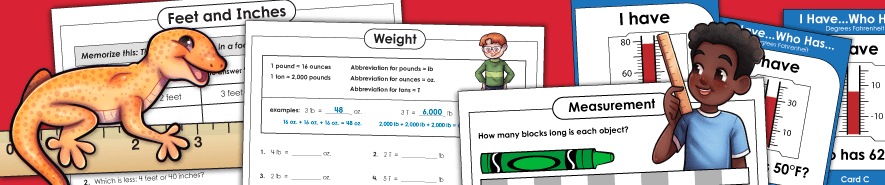# Measurement Worksheets## Linear Measurement

Linear Measurement: Standard Units
(Inches, Feet, Yards, Miles)

Measure to the nearest inch, half inch, quarter inch, eighth inch. Convert between feet, inches, and yards.

Linear Measurement: Metric Units
(Millimeters, Centimeters, Meters, Kilometers)

Measure to the nearest centimeter or millimeter. Convert between millimeters, centimeters, meters, and kilometers.

Linear Measurement: Non-Standard Units

Use non-standard units to measure objects. (example: How many blocks long is the snake?) Also includes worksheets that use words longer, shorter, taller, and smaller.

## Liquid Measurement or Capacity

Capacity: Standard Units
(Gallons, Quarts, Liters, Cups, Pints)

Measure using gallons, quarts, pints, and cups. Many worksheets include GallonBot reference illustrations.

Capacity: Metric Units
(Milliliters and Liters)

Measure using milliliter and liter metric units. Convert between milliliters and liters.

On these worksheets, students must measure the volume of the liquids shown in the graduated cylinders.

## Mass or Weight Measurement

Weight: Standard Units
(Pounds, Ounces, Tons)

Find weight measurements in customary units (ounces, pounds, and tons). Convert between ounces, pounds, and tons.

Weight: Metric Units
(Grams, Kilograms)

Find weight measurements in metric units (kilograms and grams). Convert between units.

## Temperature

Temperature / Thermometers

Thermometers worksheets in Celsius and Fahrenheit; Measure to the nearest degree

Area Worksheets

Find the areas of the rectangles, triangles, trapezoids, parallelograms, and circles.

Angle Worksheets (Measurement; Types)

On these worksheets, students will use a protractor to determine the measurement of angle. They'll also learn types of angles (acute, obtuse, right, etc.).

Fraction Worksheets

This page has lots of basic fraction worksheets. Identify fractions of a shape, as well as fractions of a set. Learn the difference between numerators and denominators.

Perimeter Worksheets

On these printables, students will add the lengths of the sides of the polygons to find the perimeters.